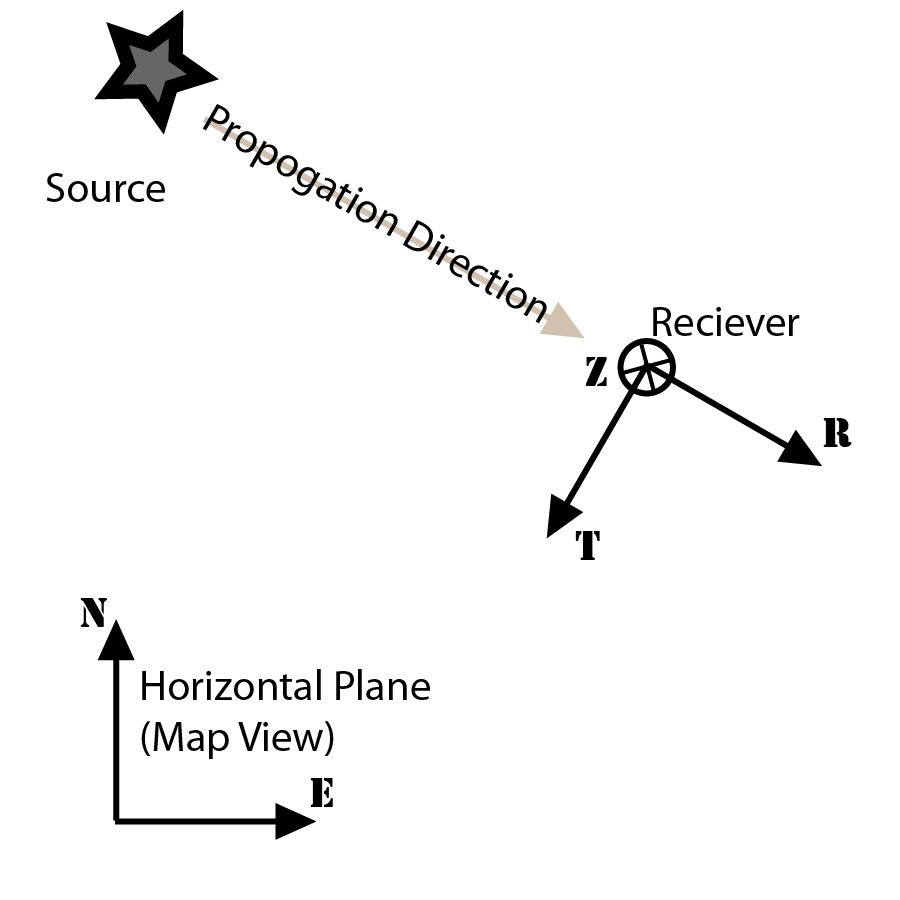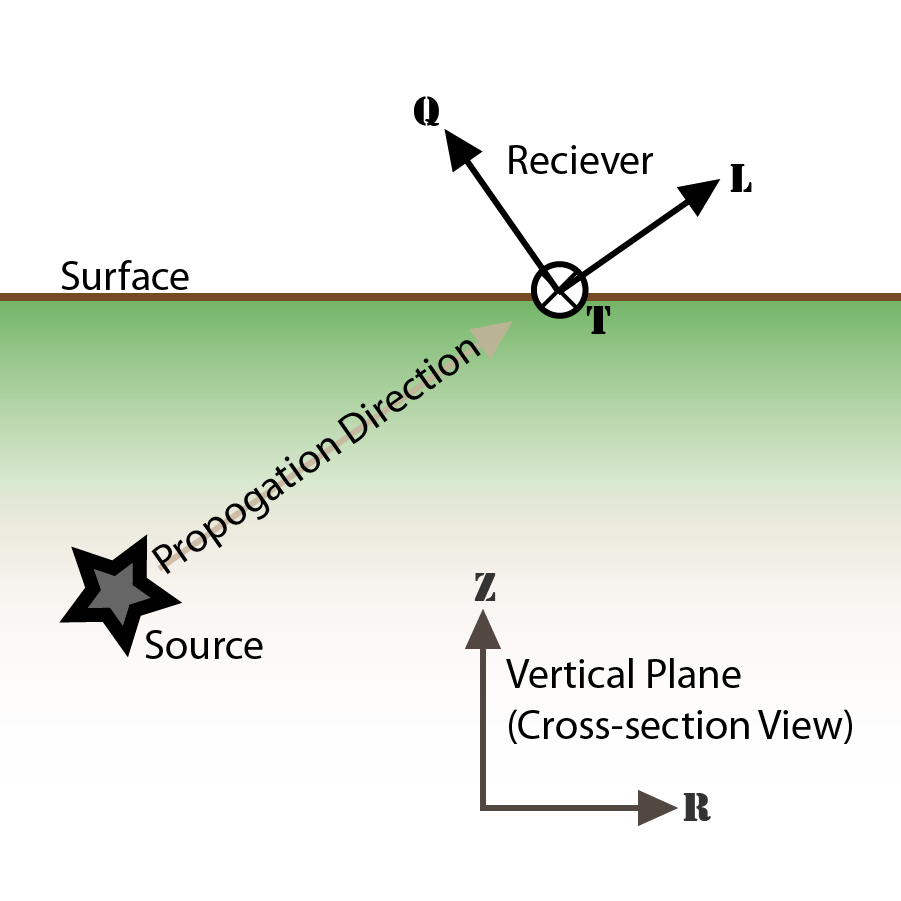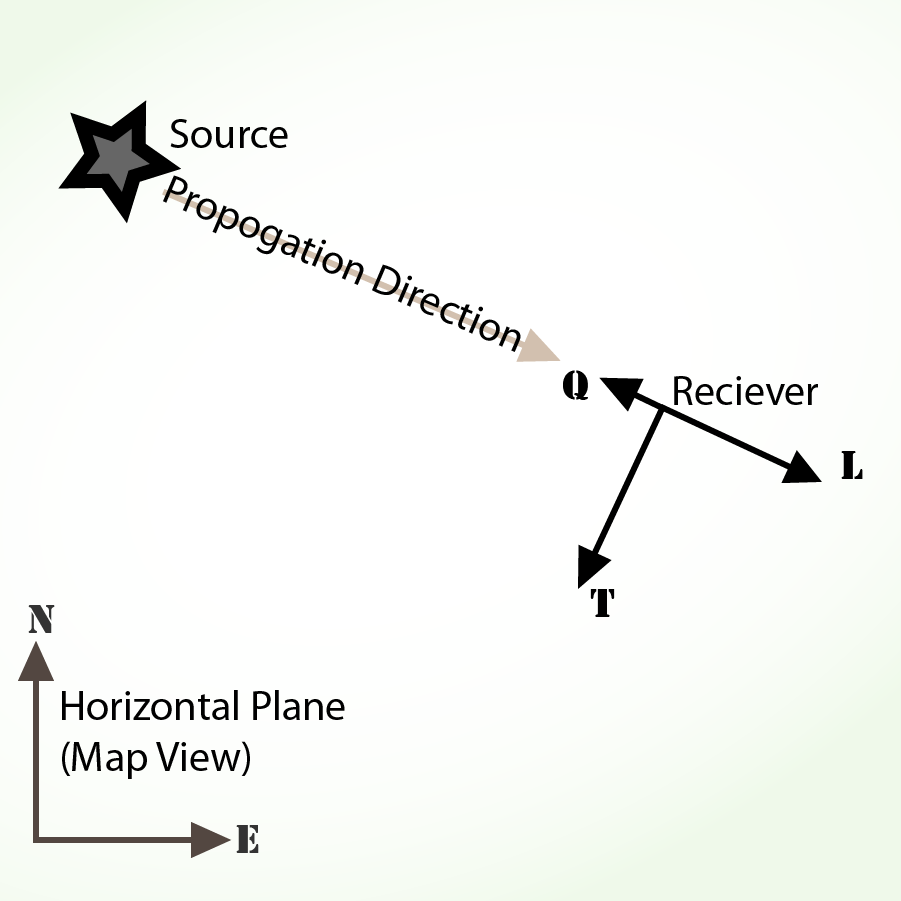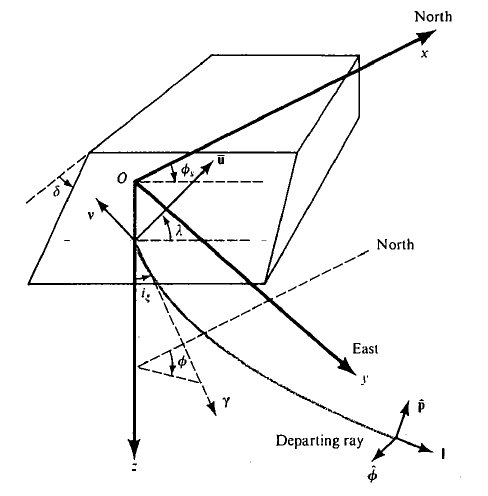三分量波形数据坐标系

NEZ坐标系

NEZ 是最简单也是最常见的坐标系。

• N: 正北方向为正方向
• E: 正东方向为正方向
• Z: 垂直向上为正方向

RTZ坐标系

RTZ的定义如下：

• R: R方向定义为从震中位置沿着大圆路径指向台站的方向
• T: T方向垂直于R和Z方向所构成的平面，且选取的T轴正方向使得RTZ构成了左手坐标系
• Z: 垂直向上为正方向NEZ坐标系到RTZ坐标系的转换公式为

$\begin{bmatrix} R \\T \end{bmatrix}$ = $\begin{bmatrix} \cos\alpha & \sin\alpha \\ -\sin\alpha & \cos\alpha \end{bmatrix}$ $\begin{bmatrix} N \\ E \end{bmatrix}$

LQT坐标系

LQT坐标系的定义如下：

• L: P波偏振方向
• Q: SV波偏振方向
• T: SH波偏振方向，与 RTZ 坐标系中T的定义相同地震矩张量张量坐标系

$\begin{bmatrix} M_{xx} & M_{xy} & M_{xz} \\ M_{yx} & M_{yy} & M_{yz} \\ M_{zx} & M_{zy} & M_{zz} \end{bmatrix}$

$\begin{bmatrix} M_{xx} & M_{xy} & M_{xz} \\ M_{xy} & M_{yy} & M_{yz} \\ M_{xz} & M_{yz} & M_{zz} \end{bmatrix}$

Mxx Myy Mzz Mxy Mxz Myz

NED 坐标系

NED 坐标系在 Aki & Richards (1980) 中有详细定义，即：

• X=North
• Y=East
• Z=DownUSE/RTP 坐标系

USE 坐标系的定义为:

• X=Upper
• Y=South
• Z=East

USE 坐标系还有很多别名，比如：

• GlobalCMT 所使用的坐标系称为RTP坐标系，RTP坐标系其实就是USE坐标系。

• X=R=Upper
• Y=T=South
• Z=P=East
• 在GMT的psmeca中，RTP坐标系也称为RTF坐标系

• 在Aki&Richards(1980)中，RTP坐标系写作 $r\Delta\phi$ 坐标系

Aki&Richards (1980) P118 给出了USE/RTP坐标系与NED坐标系之间的转换关系：

$\begin{bmatrix} M_{rr} & M_{rt} & M_{rp} \\ M_{tr} & M_{tt} & M_{tp} \\ M_{pr} & M_{pt} & M_{pp} \end{bmatrix}$=$\begin{bmatrix} M_{dd} & M_{dn} & -M_{de} \\ M_{nd} & M_{nn} & -M_{ne} \\ -M_{ed} & -M_{en} & M_{ee} \end{bmatrix}$